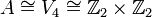# Second cohomology group for trivial group action of E8 on V4

This article gives information about the second cohomology group for trivial group action (i.e., the second cohomology group with trivial action) of the group elementary abelian group:E8 on Klein four-group. The elements of this classify the group extensions with Klein four-group in the center and elementary abelian group:E8 the corresponding quotient group. Specifically, these are precisely the central extensions with the given base group and acting group.
The value of this cohomology group is elementary abelian group:E4096.
Get more specific information about elementary abelian group:E8 |Get more specific information about Klein four-group|View other constructions whose value is elementary abelian group:E4096

## Description of the group

This group is defined as the second cohomology group for trivial group action of elementary abelian group:E8 on Klein four-group, i.e., the group:$\! H^2(G,A)$

where$G \cong E_8 \cong \mathbb{Z}_2 \times \mathbb{Z}_2 \times \mathbb{Z}_2$ and$A \cong V_4 \cong \mathbb{Z}_2 \times \mathbb{Z}_2$.

The group is an elementary abelian group of order$2^{12} = 4096$.

## Elements

Cohomology class type Number of cohomology classes Corresponding group extension GAP ID (second part, order is 32) Base characteristic in whole group?
trivial 1 elementary abelian group:E32 51 No
symmetric nontrivial direct product of Z4 and Z4 and Z2 21 Yes
symmetric nontrivial direct product of E8 and Z4 45 No
non-symmetric direct product of SmallGroup(16,3) and Z2 22 Yes
non-symmetric direct product of SmallGroup(16,4) and Z2 23 Yes
non-symmetric SmallGroup(32,24) 24 Yes
non-symmetric direct product of D8 and Z4 25 Yes
non-symmetric direct product of Q8 and Z4 26 Yes
non-symmetric SmallGroup(32,27) 27 Yes
non-symmetric SmallGroup(32,28) 28 Yes
non-symmetric SmallGroup(32,29) 29 Yes
non-symmetric SmallGroup(32,30) 30 Yes
non-symmetric SmallGroup(32,31) 31 Yes
non-symmetric SmallGroup(32,32) 32 Yes
non-symmetric SmallGroup(32,33) 33 Yes
non-symmetric SmallGroup(32,34) 34 Yes
non-symmetric SmallGroup(32,35) 35 Yes
non-symmetric direct product of D8 and V4 46 No
non-symmetric direct product of Q8 and V4 47 No
non-symmetric direct product of SmallGroup(16,13) and Z2 48 Yes